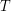## CVaR hedging using quantization based stochastic approximation algorithm

 Title CVaR hedging using quantization based stochastic approximation algorithm Publication Type Journal Article Year of Publication 2010 Authors Olivier Bardou, Noufel Frikha, and Gilles Pagès Keywords CVaR, quantization, Robbins-Monro algorithm, stochastic approximation, VaR Abstract In this paper, we investigate a method based on risk minimization to hedge observable but non-tradable source of risk on financial or energy markets. The optimal portfolio strategy is obtained by minimizing dynamically the Conditional Value-at-Risk (CVaR) using three main tools: stochastic approximation algorithm, optimal quantization and variance reduction techniques (importance sampling (IS) and linear control variable (LCV)) as the quantities of interest are naturally related to rare events. As a first step, we investigate the problem of CVaR regression, which corresponds to a static portfolio strategy where the number of units of each tradable assets is fixed at time 0 and remains unchanged till time. We devise a stochastic approximation algorithm and study its a.s. convergence and rate of convergence. Then, we extend to the dynamic case under the assumption that the process modelling the non-tradable source of risk and financial assets prices are Markov. Finally, we illustrate our approach by considering several portfolios in the incomplete energy market.
AttachmentSize
CVaR_hedging.pdf369.08 KB